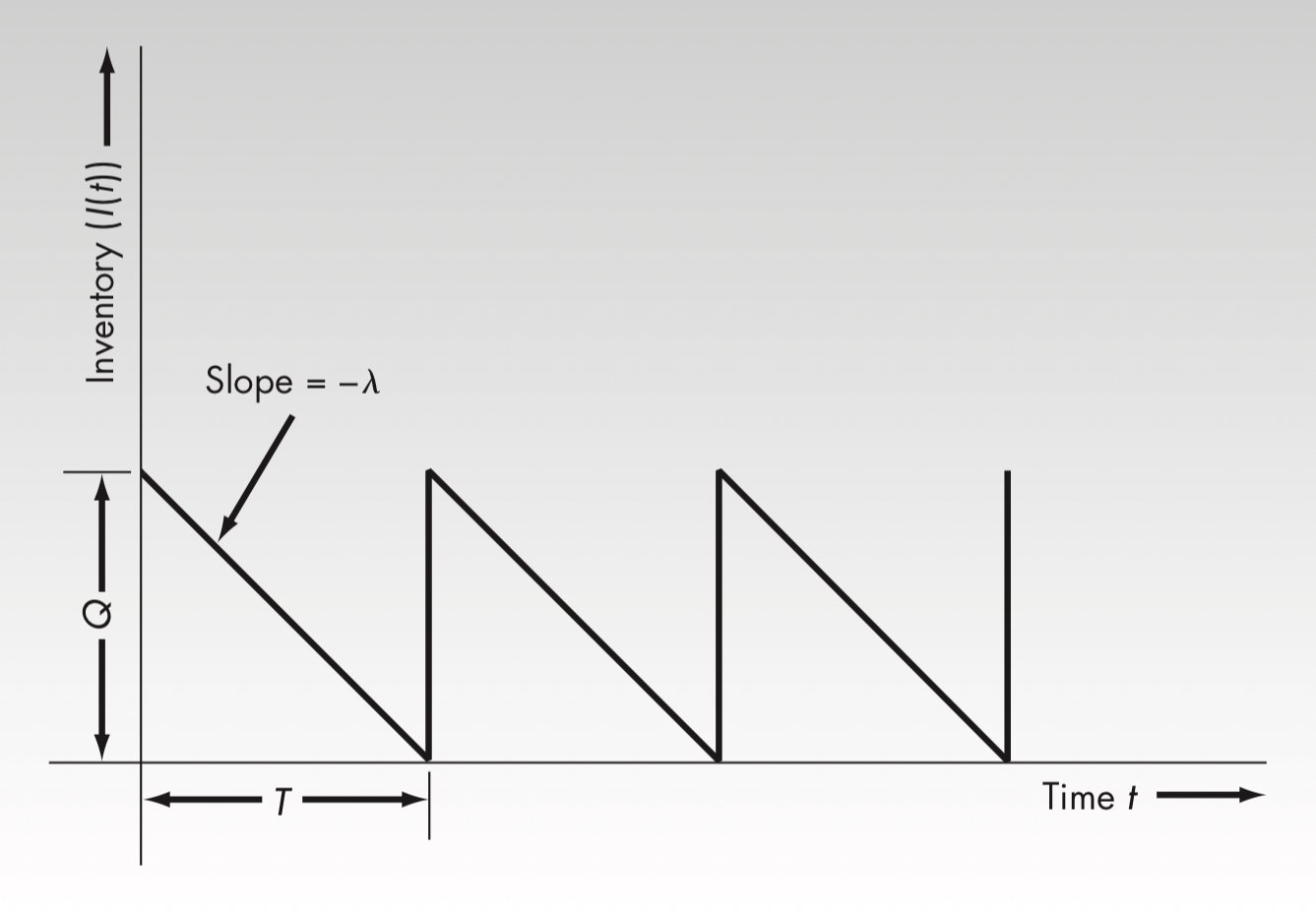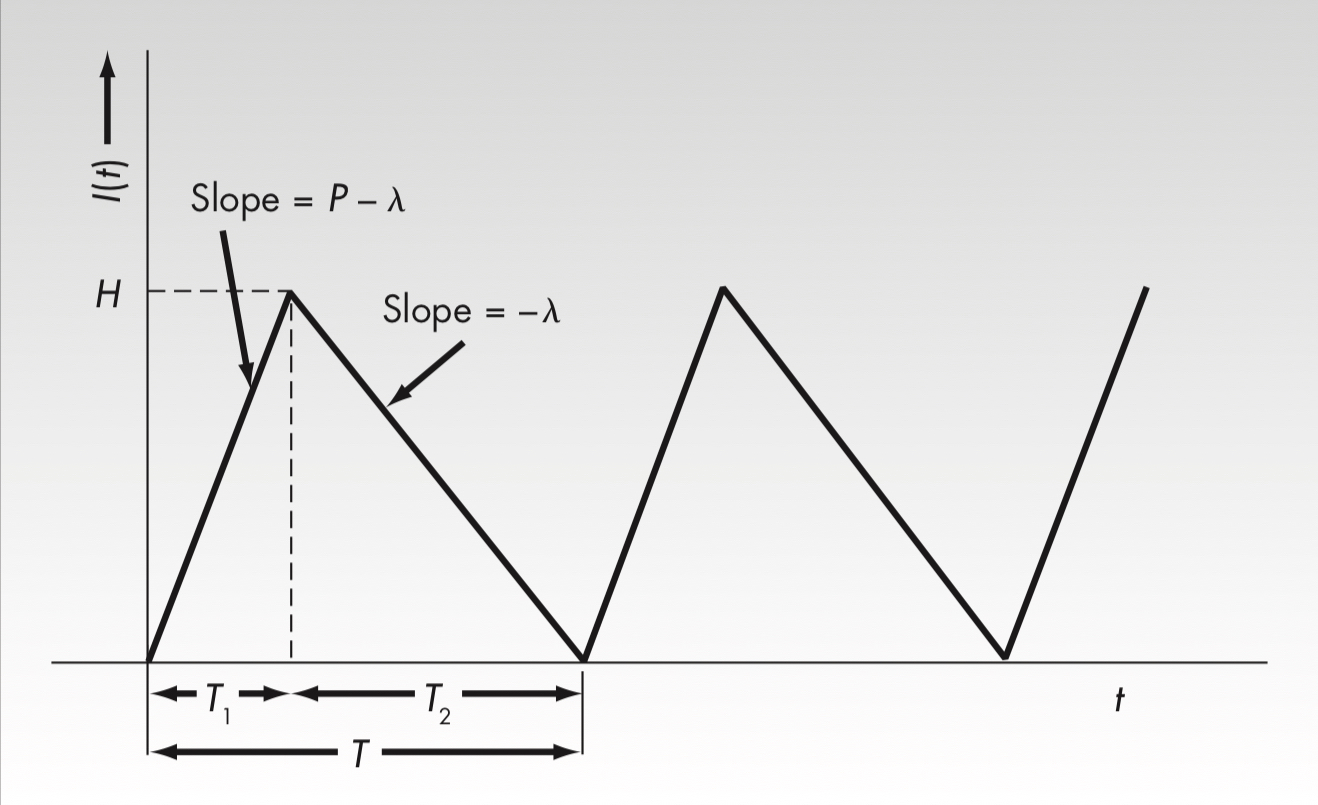# Inventory Control Subject to Known Demand 1

Inventory Control Subject to Known Demand 1Economic Order Quantity ModelThis is the simplest model based on totally known constant demand rate on time. Though simple, it captures the essence of inv...

# Inventory Control Subject to Known Demand 1

## Economic Order Quantity Model

This is the simplest model based on totally known constant demand rate on time. Though simple, it captures the essence of inventory control—tradeoff between lasting holding cost and one-off setup cost.

#### Notation

$K$, setup cost,
$c$, unit cost,
$i$, annual inventory holding cost rate,
$\lambda$, annual demand or demand rate in the scope of year,
$Q$, order quantity each order,
$\tau=0$, no lead time for every order.

The policy is to place an order $Q$ every time when inventory hits zero.Total cost function (yearly),

$G(Q)=K\frac{\lambda}{Q}+ic\frac{Q}{2}+(c\lambda)$

First term—setup cost, for every year’s demand we need to order $\lambda/Q$ times, each setup costs $K$.
Second term—holding cost, average inventory is $Q/2$ because demand is constant and linear on time. Multiplying it by annual rate and unit cost can we get the yearly holding cost.
Third term—unit cost, here we assume that all demands are satisfied and thus the choice of $Q$ doesn’t influence the exogenous total unit cost, which is why I bracket the third term.
To minimize total cost,

$min\quad G(Q)=K\frac{\lambda}{Q}+ic\frac{Q}{2}$
First order condition,

$G’(Q)=-K\frac{\lambda}{Q^2}+\frac{ic}{2}=0$

Solution,

$Q^*=\sqrt{\frac{2K\lambda}{ic}}$

#### Sensitivity Analysis

Though EOQ can be derived easily, it is generally much more complicated when applying EOQ policy in real life due to various constraints on order quantity. However, one can compare the cost between EOQ and alternate order quantities by a practical formula,

\begin{aligned} \frac{G(Q)}{G(Q^*)}&=\frac{K\lambda/Q+icQ/2}{K\lambda/Q^*+icQ^*/2}\\\\&=\frac{K\lambda/Q+icQ/2}{\sqrt{icK\lambda/2}+\sqrt{K\lambda ic/2}}\\\\&=\frac{K\lambda Q+icQ/2}{\sqrt{2K\lambda ic}}\\ \\ &=\frac{\frac{2K\lambda}{ic}/Q+Q}{2\sqrt{\frac{2K\lambda}{ic}}}\\\\&=\frac{Q^{*2}/Q+Q}{2Q^*}\\ \\&=\frac{1}{2}[\frac{Q^*}{Q}+\frac{Q}{Q^*}]. \end{aligned} Note that the cost excludes unit cost.

## Economic Production Quantity

Sometimes producers don’t order externally but produce internally, in which inventory needs some time to increase.We have cost function,

$G(Q)=K\frac{\lambda}{Q}+ic\frac{H}{2}+(c\lambda)$

Although we don’t know $H$, the production and the consumption should be equal,

$PT_1=\lambda T=Q$
$T_1=\frac{Q}{P}$

Thus,

$H=(P-\lambda)T_1=(P-\lambda)\frac{Q}{P}$

Plug $H$ in we can get,

$G(Q)=K\frac{\lambda}{Q}+ic\frac{(P-\lambda)Q/P}{2}+(c\lambda)$

First order condition,

$G’(Q)=-K\frac{\lambda}{Q^2}+\frac{ic}{2}(1-\frac{\lambda}{P})=0$ $Q^*=\sqrt{\frac{2K\lambda}{ic(1-\lambda/P)}}$